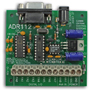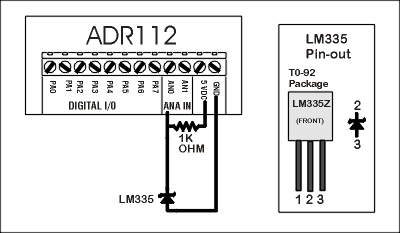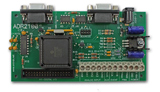Solid State Temperature Measurement The LM335 solid-state temperature sensor produces an output of 10mV per degree Kelvin. Kelvin can be converted to Celsius by subtracting 273 or 2.73 volts from the reading.   The sensors high sensitivity allow it to be used with 10-bit or 12-bit analog inputs  directly with only the use of a 1K ohm bias resistor. The following diagram shows the connection of an LM335  to an ADR112which features 12-bit analog inputs.  The reading can be converted to Celsius using the following formula and resolution is approx.  0.1 degrees Celsius; C= ( reading-2235.9)/ 8.1910 OPEN "COM1:9600,N,8,1,CS,DS,RS" AS#1 20 CLS 30 LOCATE 1,1 40 PRINT#1, "RD0" 50 INPUT#1, READING 60 CEL= ( READING/2235.9)/8.19 70 PRINT " TEMPERATURE IS " CEL, "DEGREES CELCIUS" 80 GOTO 30      ADR2100analog input resolution is 10-bits and allows a resolution of   0.5 Degrees Celcius. The following formula is used to calculate temperature in Celsius. C= ( reading-558.6)/ 2.048 ADR101analog input resolution is  8-bits   and allows a resolution of  2 degrees C. The following formula is used to calculate temperature in Celsius. C= ( reading-139.2 )/0.512 ( NOTE : The LM335 sensor can be purchased from Digi-Key as part # LM335AZ-ND. Their phone number is 1-800-344-4539 and costs around \$5 U.S. Digi-Key accepts all major credit cards and the part is always in stock.) Back to Applications Page